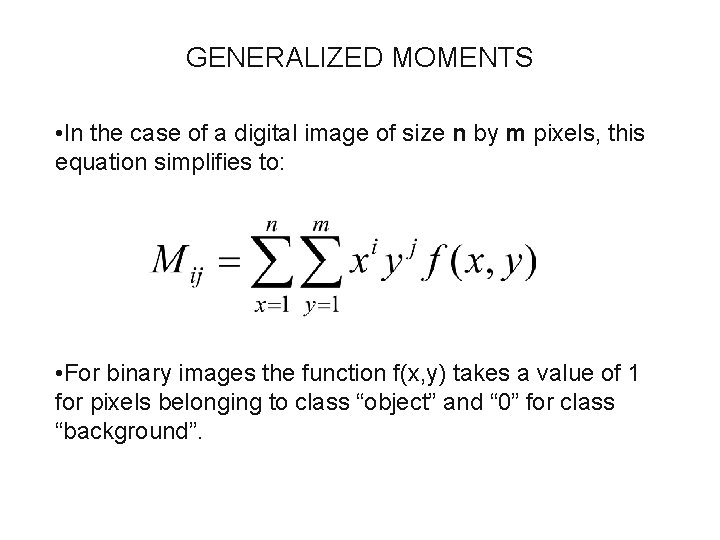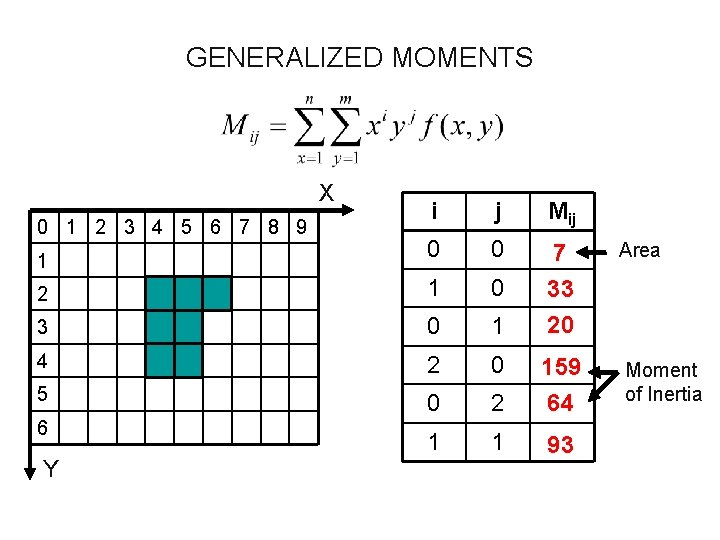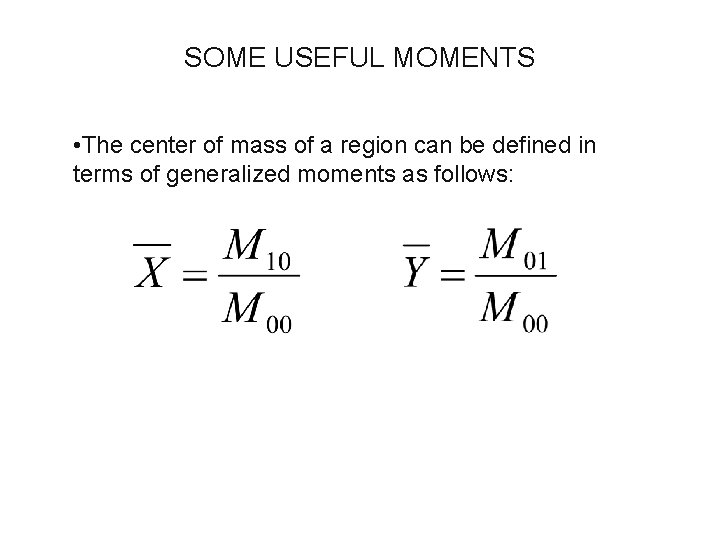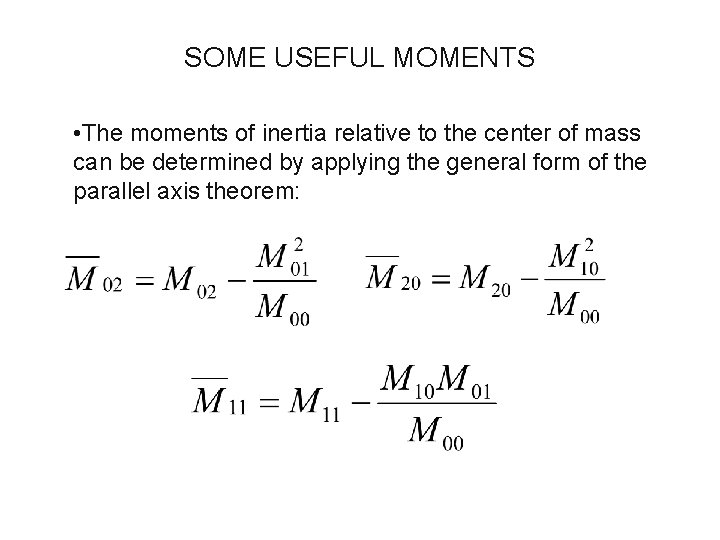# OBJECT RECOGNITION BLOB ANALYSIS Examples of form parameters

• Slides: 12
Download presentationOBJECT RECOGNITION – BLOB ANALYSIS • Examples of form parameters that are invariant with respect to position, orientation, and scale: • Number of holes in the object • Compactness or Complexity: (Perimeter)2/Area • Moment invariants • All of these parameters can be evaluated during contour following.GENERALIZED MOMENTS • Shape features or form parameters provide a high level description of objects or regions in an image • Many shape features can be conveniently represented in terms of moments. The (p, q)th moment of a region R defined by the function f(x, y) is given by:GENERALIZED MOMENTS • In the case of a digital image of size n by m pixels, this equation simplifies to: • For binary images the function f(x, y) takes a value of 1 for pixels belonging to class “object” and “ 0” for class “background”.GENERALIZED MOMENTS X i j Mij 1 0 0 Area 2 1 0 3 0 1 7 33 20 4 2 0 5 0 2 159 64 Moment of Inertia 1 1 93 0 1 2 3 4 5 6 7 8 9 6 YSOME USEFUL MOMENTS • The center of mass of a region can be defined in terms of generalized moments as follows:SOME USEFUL MOMENTS • The principal axis of an object is the axis passing through the center of mass which yields the minimum moment of inertia. • This axis forms an angle θ with respect to the X axis. • The principal axis is useful in robotics for determining the orientation of randomly placed objects.Principal Axis: DerivationExample X Principal Axis Center of Mass YSOME USEFUL MOMENTS • The moments of inertia relative to the center of mass can be determined by applying the general form of the parallel axis theorem:SOME (MORE) USEFUL MOMENTS • The minimum/maximum moment of inertia about an axis passing through the center of mass are given by:SOME (MORE) USEFUL MOMENTS • The following moments are independent of position, orientation, and reflection. They can be used to identify the object in the image.SOME (MORE) USEFUL MOMENTS • The following moments are normalized with respect to area. They are independent of position, orientation, reflection, and scale.